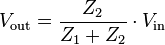# How to sense 12v or Higher with Arduino?

Hi all,

My question is regarding the "How to sense 12v or Higher Circuitry with Arduino" circuit found on the Arduino Playground. The title of the PDF is Level_Shifting so I assume that is what it is, a level shifter?

I have built it and it works but to be honest I can't remember the maths to justify the results. Can anyone give a quick breakdown of how this works and possible what the ratios of the resistor values means?

Just use a voltage divider:-

for full maths

I did think about that but seeing the circuit that they have put together I assumed that there was something else going on that needed to be taken into account.

I guess I thought that a voltage divider was just to simple, but it is exactly what needs to be done.

Anyone have any comments against using a simple voltage divider?

Two reasons to dislike the simple voltage divider:

1. No protection from overvoltage on the input. If you suddenly get 24V rather than 12V, your arduino is toast.

2. Voltage at the arduino pin is dependent on the actual values of the resistors and the input impedance. This can cause various sorts of trouble at the input pin.

Whether these are a bigger deal than the wonderful simplicity of the voltage divider depends on requirements.An optoisolator is another option.

You could probably use a zener diode and a resistor or two, but the exact circuit doesn't spring to mind.

-j

Thanks rocket, do you have any insight on the original question as to what the values of the resistors mean or how to calculate the values so that I can be sure that the results are making sense?

Thanks

The resistor values are proportional: e.g. using two 1k ohm resistors will drop the voltage by half between the two resistors.

Here are some factors you must consider using a voltage divider to trigger a TTL input from a voltage source Vsense:

• how much current you draw from Vsense. Ohms law (V=IR, voltage = current * resistance) is your friend: I=Vsense/(R1+R2) Too little current and you can't drive the input; too much, and you waste your battery with the divider (can even fry resistors dumping too much power if the resistor values and wattages are too small). Rule of thumb: use resistors in the thousands or tens of thousands of ohms (4.7k + 15k for a ~1/4 divider, etc)

• Vin max on the TTL input (5V on a 5V arduino). Higher than this and you damage the input pin, or even the entire device.

• Vin high min on the TTL input (5V * 0.7 = 3.5V on the arduino). Lower than this and you won't reliably trigger a digital high level on the input.

• range of Vsense. e.g., vehicle voltage can vary from 14.5V (or even higher) down to 11V or lower. You want to divide this so that it's between Vin max and Vin high min to provide safe and sane operation. Do some math to see if it's even possible.
Again, this is for a TTL input. If you're using an ADC input, the rules are a bit easier (just don't go over Vin max on the ADC input) and you take care of the rest in software.

-j

No protection from overvoltage on the input.

so see:-

http://www.thebox.myzen.co.uk/Tutorial/Protection.html

It's that simple. I tested a 1300VDC Voltage multiplier on my 200V DVM with a little math and just pair of resistors.

pwillard, could you be so kind as to share the math with some of the numbers that you used.

ThanksHere is an example of level-switching - my implemention of an autoselect 2 / 10v voltmeter on the Arduino:

![](http://quantsuff.com/images/Arduino V-meter.jpg)

``````long readV(int inPort)
/*
* Returns Volts at inPort in milliVolts
* Aref must be set to "INTERNAL" for these conversions to work!
* Warning: The 5v aref may not be accurate with USB!
* (c) 2009 qs@quantsuff.com
*/
{
long V ;
* then mult the previous # to get new adjustment.
* 1M in series acts as V-divider
* Parallel 150k to gnd to get 9.5v fs */

float ref9V5adjust = 9.22983;  // Start: 9.319;
float ref2V2adjust = 2.121742; //Cal'd against known good meter

digitalWrite(13,HIGH) ; // 10v scale first
delay(10);
V= analogRead(inPin); // 0-1023 on 2v2 scale